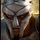Error in Blog Post Code

Found this confidence interval post very helpful, but the code didn't' work properly for me until I changed the 'rates' table name to 'conversion' in the outermost SELECT statement:

```select
rates.n as users,
rates.x as conversions,
intervals.n as users,
intervals.x as conversions,
p - se * 1.96 as low,
intervals.p as mid,
p + se * 1.96 as high
from (
select
rates.*,
sqrt(p * (1 - p) / n) as se -- calculate se
from (
select
conversions.*,
(x + 1.92) / (n + 3.84)::float as p -- calculate p
from (
-- Our conversion rate table from above
select
count(1) as n,
sum(case when converted then 1 else 0 end) as x
from users
group by date_trunc('month', created_at);
) conversions
) rates
) intervals```

Anyone else experience this issue?